# Civil Engineering - GATE Exam Questions

### Exercise :: GATE Exam Questions - Section 1

26.

A cantilever beam of length L and a cross section with shape factor f supports a concentrated load P as shown below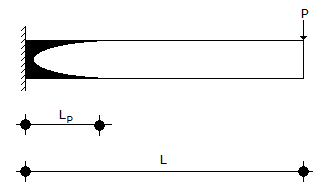The length LP of the plastic zone, when the maximum bending moment equals the plastic moment MP, given by

 A.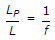B.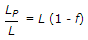C.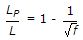D.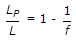Explanation:

No answer description available for this question. Let us discuss.

27.

The minimum area of tension reinforcement in a beam shall be greater than

 A. 0.85 bd/fy B. 0.87 fy/bd C. 0.04 bd D. 0.4 bd/y

Explanation:

No answer description available for this question. Let us discuss.

28.

In an iceberg, 15% of the volume projects above the sea surface. If the specific weight of sea water is 10.5 kN/m3 , the specific weight of iceberg ir kN/m3 is

 A. 12.52 B. 9.81 C. 8.93 D. 7.83

Explanation:

No answer description available for this question. Let us discuss.

29.

A reinforced concrete column contains longitudinal steel equal to 1 percent of net cross-sectional area of the column. Assume modular ratio as 10. The loads carried (using the elastic theory) by the longitudinal steel and the net area of concrete, are Ps and Pc respectively. The ratio Ps/Pc expressed as percent is

 A. 0.1 B. 1 C. 1.1 D. 10

Explanation:

No answer description available for this question. Let us discuss.

30.

A steady discharge of 1 cumec flows uniformly in a rectangular channel 1 m wide at a depth of 250 mm. The slope of the channel bed is :

 A. adverse B. steep C. critical D. mild